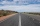# Circle chord

What is the length d of the chord circle of diameter 36 m, if distance from the center circle is 16 m?

Result

d =  16.5 m

#### Solution:Leave us a comment of example and its solution (i.e. if it is still somewhat unclear...):

Showing 0 comments:Be the first to comment!#### To solve this example are needed these knowledge from mathematics:

Pythagorean theorem is the base for the right triangle calculator. See also our trigonometric triangle calculator.

## Next similar examples:

1. RectangleThe rectangle is 11 cm long and 45 cm wide. Determine the radius of the circle circumscribing rectangle.
2. Tetrahedral pyramidWhat is the surface of a regular tetrahedral (four-sided) pyramid if the base edge a=7 and height v=6?
3. MovementFrom the crossing of two perpendicular roads started two cyclists (each at different road). One runs at average speed 28 km/h, the second at average speed 24 km/h. Determine the distance between them after 45 minutes cycling.
4. Right ΔRight triangle has length of one leg 28 cm and length of the hypotenuse 53 cm. Calculate the height of the triangle.
5. RiverCalculate how many promiles river Dunaj average falls, if on section long 957 km flowing water from 1454 m AMSL to 101 m AMSL.
6. Prism 4 sidesThe prism has a square base with a side length of 3 cm. The diagonal of the sidewall of the prism/BG/is 5 cm. Calculate the surface of this prism in cm square and the volume in liters
7. GardenArea of square garden is 6/4 of triangle garden with sides 56 m, 35 m and 35 m. How many meters of fencing need to fence a square garden?
8. Castle towerThe castle tower has a cone-shaped roof with a diameter of 10 meters and a height of 8 meters. Calculate how much m² of coverage is needed to cover it if we must add one-third for the overlap.
9. Pile of sandA large pile of sand has been dumped into a conical pile in a warehouse. The slant height of the pile is 20 feet. The diameter of the base of the sand pile is 31 feet. Find the volume of the pile of sand.
10. Horizontal distanceThe road has a gradient of 8%. How many meters will the road rise on a horizontal distance of 400m?
11. Diamond and diagonalsA diamond has diagonals f = 8 cm and g = 6 cm long. How long is this diamond perimeter? (Calculate it!)
12. The sides 2The sides of a trapezoid are in the ratio 2:5:8:5. The trapezoid’s area is 245. Find the height and the perimeter of the trapezoid.
13. CincinnatiA map is placed on a coordinate grid. Cincinnati located at (5,4) and San Diego is located at (-10, -3). How far apart is Cincinnati from San Diego on the map? Round to the nearest tenth.
14. Axial sectionAxial section of the cone is equilateral triangle with area 208 dm2. Calculate volume of the cone.
15. Triangle SASCalculate area and perimeter of the triangle, if the two sides are 51 cm and 110 cm long and angle them clamped is 130°.
16. SquarePoints A[-5,-6] and B[7,-1] are adjacent vertices of the square ABCD. Calculate the area of the square ABCD.
17. Is right?Is triangle with sides 51, 56 and 77 right triangle?